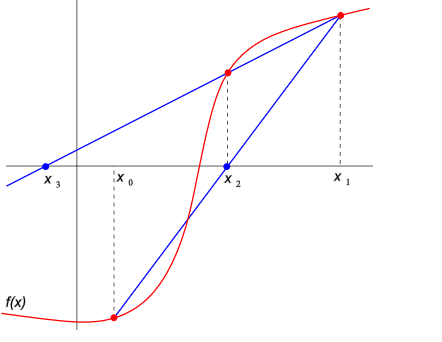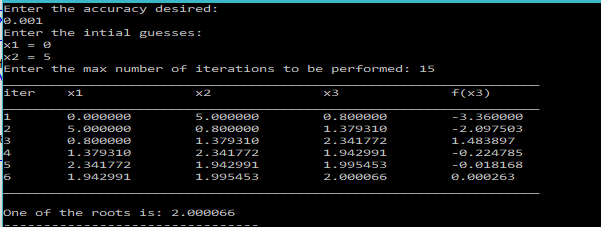# Secant Method – C Program

Secant Method, is a Numerical Technique to find the root of an algebraic or transcendental equation.
The root is approximated by drawing secant lines repeatedly.A secant line is a line joining two points on a function. Secant method requires two initial guesses(x0 and x1), to draw the first secant line. The root of this line(x2), that is, where this line touches the x-axis, becomes the new point, and now a secant line is drawn between the new point(x2) and one of the last points(x1).
This process is repeated until a root is found upto a certain tolerance.

The method is similar to Bisection Method, in that it requires two initial guesses, but still a lot different, as the guesses don’t require to bracket(enclose) the root. Moreover, unlike Bisection Method, Secant Method may not always converge, so it might be a good idea to have a limit for the maximum iterations to be performed.

So, the program would ask the user to enter two initial guesses:x1 and x2.
Then, it will calculate the new point(x3) using the formula:$x_3=\frac{x_1f(x_2)-x_2f(x_1)}{f(x_2)-f(x_1)}$

### PROGRAM (Simple Version):

/***********************************
*********SECANT METHOD**************
***********************************/
#include<stdio.h>
#include<math.h>
/*Function whose root is to be determined*/
double f(double x){
return x*x-4;
}
main(){
int iter=1,maxSteps;
double x1,x2,x3,eps;
printf("Enter the accuracy desired: \n");
scanf("%lf",&eps);
printf("Enter the intial guesses: \nx1 = ");
scanf("%lf",&x1);
printf("x2 = ");
scanf("%lf",&x2);
printf("Enter the max number of iterations to be performed: ");
scanf("%d",&maxSteps);
printf("iter\tx1\t\tx2\t\tx3\t\tf(x3)\n");
printf("___________________________________________________________________\n");
do{
x3=(x1*f(x2)-x2*f(x1))/(f(x2)-f(x1));
printf("%d\t%lf\t%lf\t%lf\t%lf\n",iter,x1,x2,x3,f(x3));
x1=x2;
x2=x3;
iter++;
}while(fabs(f(x3))>eps&&iter<=maxSteps);
printf("\nOne of the roots is: %lf",x3);
}

### PROGRAM (USING FUNCTIONS)

/***********************************
*********SECANT METHOD**************
***********************************/
#include<stdio.h>
#include<math.h>
/*Function whose root is to be determined*/
double f(double x){
return x*x-4;
}
/*Function that returns the root from Secant Method*/
double secant(double f(double x), double x1, double x2, double eps, int maxSteps){
int iter=1;
double x3;
do{
x3=(x1*f(x2)-x2*f(x1))/(f(x2)-f(x1));
x1=x2;
x2=x3;
iter++;
}while(fabs(f(x3))>eps&&iter<=maxSteps);
return x3;
}
/*Secant Method Function that tabulates the values at each iteration*/
double secantPrint(double f(double x), double x1, double x2, double eps, int maxSteps){
int iter=1;
double x3;
printf("___________________________________________________________________\n");
printf("iter\tx1\t\tx2\t\tx3\t\tf(x3)\n");
printf("___________________________________________________________________\n");
do{
x3=(x1*f(x2)-x2*f(x1))/(f(x2)-f(x1));
printf("%d\t%lf\t%lf\t%lf\t%lf\n",iter,x1,x2,x3,f(x3));
x1=x2;
x2=x3;
iter++;
}while(fabs(f(x3))>eps&&iter<=maxSteps);
printf("___________________________________________________________________\n");
return x3;
}
main(){
int maxSteps;
double x1,x2,x3,eps;
printf("Enter the accuracy desired: \n");
scanf("%lf",&eps);
printf("Enter the intial guesses: \nx1 = ");
scanf("%lf",&x1);
printf("x2 = ");
scanf("%lf",&x2);
printf("Enter the max number of iterations to be performed: ");
scanf("%d",&maxSteps);
printf("\nOne of the roots is: %lf",secantPrint(f,x1,x2,eps,maxSteps));
}


### OUTPUT:[wpedon id="7041" align="center"]